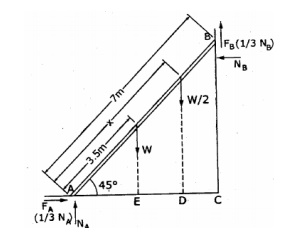Home | | Engineering Mechanics | Important Questions and Answers: Friction and Elements of Rigid Body Dynamics

# Important Questions and Answers: Friction and Elements of Rigid Body Dynamics

Engineering Mechanics - Friction and Elements of Rigid Body Dynamics

(FRICTION AND ELEMENTS OF RIGID BODY DYNAMICS)

1.Define instantaneous centre of rotation.

Instantaneous centre of rotation is a point identified with in a body where the velocity is zero.

2.Define co-efficient of restitution.

It is ratio of magnitudes of impulses corresponding to the period of restitution and to the period of deformation is called coefficient of restitution .

3.Define kinetics.

Study of bodies subjected to forces which are unbalanced is called kinetics.

4. Define kinematics

The study of geometry and time dependent aspects of motion without considering forces causing motion.

5.Define Angular momentum.

Momentum of linear momentum is called angular momentum.

6.what is general plane motion ? give some examples.

When motion of particles  and rigid bodies defined in a plane is called

plane motion.

Example :All planets revolving around the sun.

7.Define Relative velocity.

Assume particle A moves with a velocity of Va and particle B moves with a velocity Vb.

8.Define Resultant velocity.

Assume particle A moves to x direction Vx and in y direction Vy this resultant velocity.

9.How will you calculate the linear restoring force of an elastic material.

Linear restoring force of an elastic material. F=kx

Where k is the stiffness of the material and x is the displacement.

10.state the principle of work and energy.

The principle of work and energy or work energy equation is written as Work done =final kinetis energy - initial kinetis energy

11.Define instantaneous centre of rotation.

A rigid body in plane motion can be considered to rotate about a point that remains at a particular instant. This point having zero instantaneous velocity is called the instantaneous centre of rotation.

1.Give mathematical definitions of velocity and acceleration.

2.A Car traverses half of a distance with a velocity of 40 Kmph and the remaining half of distance with a velocity of 60 Kmph. Find the average velocity.

3.Define friction and classify its types. 4.Classify the types of friction.

5.Define Limiting friction.

6.Define coefficient of static friction. 7.Stat coulomb’s laws of dry friction. 8.Define rolling resistance.

9.What is coefficient of rolling resistance?

10.Define coefficient of friction and express its relationship with angle of friction.

11.If x=3.5t3– 7 t2, determine acceleration, velocity and position of the particle, when t = 5 sec. 12.Consider a wheel rolling on a straight track. Illustrate the characteristics of general plane motion.

13.Write work energy equation of rigid body. Mention the meaning for all parameters used in the equation.

14.What us general plane motion? Give some examples. 15.Define Limiting friction.

16.Define Co-efficient of friction and angle of friction 17.Define coulomb’s laws of dry friction.

18.Define impending motion. 19.Define angle of repose 20.Define cone of friction.

21.Define the following terms i) Ladder friction. ii) Wedge friction iii) Screw friction iv) Belt friction.

1. Block (2) rests on block (1) and is attached by a horizontal rope AB to the wall as shown in fig. What force P is necessary to cause motion of block (1) to impend? The co-efficient of friction between the blocks is ¼ and between the floor and block (1) is 1/3. Mass of blocks (1)and(2) are 14kg and 9 kg respectively2. Block A weighing 1000 N rests on a rough inclined plane whose inclination to the horizontal is 45°. It is connected to another block B, weighing 3000 N rests on a rough horizontal plane by a weightless rigid bar inclined at an angle of 30° to the horizontal as shown in fig. Find the horizontal force required to be applied to the block B just to move the block A in upward direction. Assume angle of friction as 15° at all surfaces where there is sliding.3. A 7m long ladder rests against a vertical wall, with which it makes an angle of 45° and on a floor. If a man whose weight is one half that of the ladder climbs it, at what distance along the ladder will he be, when the ladder is about to slip? Take coefficient of friction between the ladder and the wall is 1/3 and that between the ladder and the floor is ½.4. In a screw jack, the pitch of the square threaded screw is 5.5 mm and means diameter is 70 mm. The force exerted in turning the screw is applied at the end of lever 210 mm long measured from the axis of the screw. If the co-efficient of friction of the screw jack is 0.07. Calculate the force required at the end of the lever to (i) raise a weight of 30 KN (ii) lower the same weight.

5. An effort of 200 N is required just to move a certain body up an inclined plane of angle 15°, the force is acting parallel to the plane. If the angle of inclination of the plane is made 20°,

the effort required being again parallel to the plane, is found to be 230 N. Find the weight of the body and coefficient of friction.

Find the force P inclined at an angle of 32° to the inclined plane making an angle of 25 degree with the horizontal plane to slide a block weighing 125 KN (i) up the inclined plane (ii) Down the inclined plane, when P = 0.5.Study Material, Lecturing Notes, Assignment, Reference, Wiki description explanation, brief detail
Engineering Mechanics : Friction and Elements of Rigid Body Dynamics : Important Questions and Answers: Friction and Elements of Rigid Body Dynamics |